Function Repository Resource:

# DisplayPowersTogether

Display multiplication and/or division of factors with the form x^y as one factor

Contributed by: Ted Ersek
 ResourceFunction["DisplayPowersTogether"][expr] displays the multiplication and/or division of factors with the form xyor Surd[x,n] as one factor. ResourceFunction["DisplayPowersTogether"][expr,assume] uses assume as assumptions.

## Details and Options

ResourceFunction["DisplayPowersTogether"] automatically combines factors at all levels of an expression.
Powers are displayed as one factor when valid under assuptions given by \$Assumptions.
ResourceFunction["DisplayPowersTogether"][expr,assume] combines assume with \$Assumptions.
CubeRoot[x] evaluates to Surd[x,3], which is affected by ResourceFunction["DisplayPowersTogether"].
The output of ResourceFunction["DisplayPowersTogether"] is a Defer object, which displays with the powers together. Evaluating the Defer object can result in powers seperating.

## Examples

### Basic Examples (4)

Combine the powers in an expression:

 In:=Out=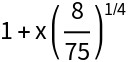Combine powers with multiple terms:

 In:=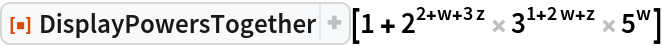Out=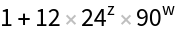In:=Out=DisplayPowersTogether also combines products and quotients that include CubeRoot:

 In:=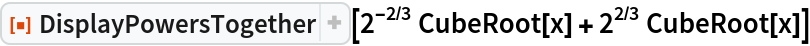Out=### Scope (9)

DisplayPowersTogether combines exponents using the default assumptions stored in \$Assumptions. The assumptions in this example are sufficient to combine all the exponents:

 In:=Out=DisplayPowersTogether similarly combines all the exponents when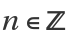is given as a second argument in DisplayPowersTogether:

 In:=Out=Provide assumptions allowing some but not all exponents to be combined:

 In:=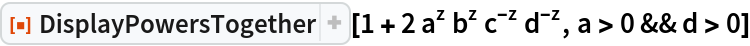Out=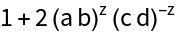Set \$Assumptions as a>0, and specify d>0 as an assumption within DisplayPowersTogether:

 In:=Out=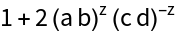Only assume d> 0:

 In:=Out=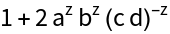The following assumptions are sufficient to combine all the exponents even though nothing is assumed about b or z:

 In:=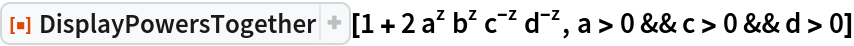Out=FullSimplify verifies that DisplayPowersTogether performed a valid transformation under the given assumptions:

 In:=Out=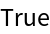This performs independent verification by searching for a counterexample to the previous example. The identity is True in every case considered:

 In:=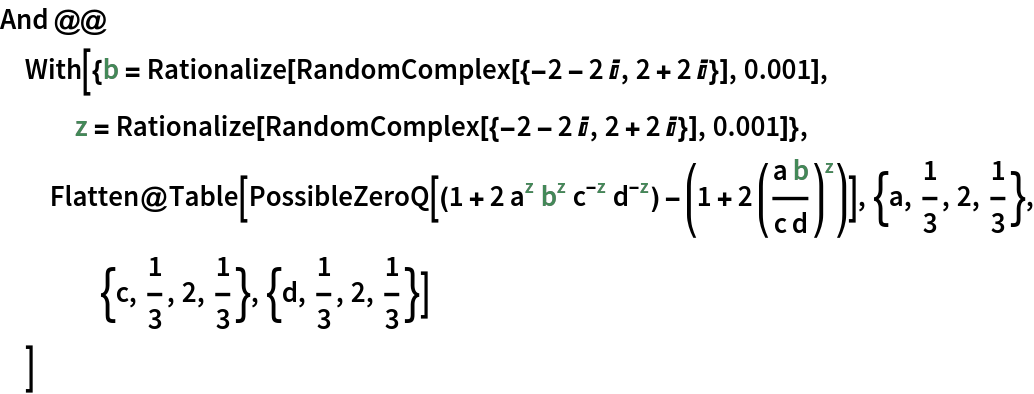Out=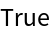In this case, nothing is assumed and none of the exponents can be combined:

 In:=Out=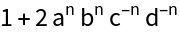DisplayPowersTogether determines this can be done with a single Sqrt:

 In:=Out=Surd is a generalization of CubeRoot. DisplayPowersTogether combines expressions involving Surd when appropriate:

 In:=Out=In:=Out=### Properties and Relations (2)

Rather than use \$Assumptions or DisplayPowersTogether[expr,assum], assumptions can be provided using Assuming:

 In:=Out=Two examples are given here where Simplify can combine a sum of terms:

 In:=Out=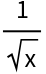In:=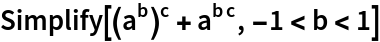Out=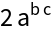DisplayPowersTogether does not handle sums or differences:

 In:=Out=In:=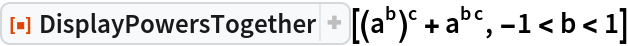Out=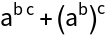### Possible Issues (2)

The result of DisplayPowersTogether has a Head of Defer:

 In:=Out=In:=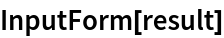Out=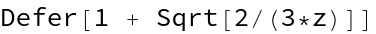It is not a readily-computable expression:

 In:=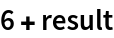Out=First can be used to compute on the results:

 In:=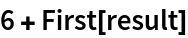Out=Alternatively, copy the output shown previously and evaluate it to perform the computation:

 In:=Out=DisplayPowersTogether can be used again to ensure the square roots are combined again:

 In:=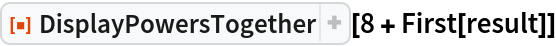Out=The next example is not in a form that allows DisplayPowersTogether to combine factors:

 In:=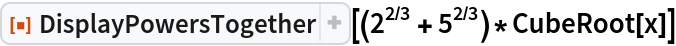Out=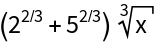When the cube root above is distributed across the sum, DisplayPowersTogether can combine factors:

 In:=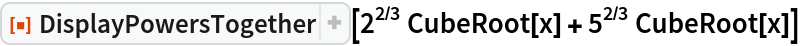Out=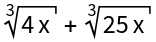Ted Ersek

## Version History

• 1.0.0 – 02 October 2020